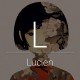### 题目链接：

http://exam.upc.edu.cn/problem.php?id=5124

## 题目：

### 题目描述

Flow Free is a puzzle that is played on a 2D grid of cells, with some cells marked as endpoints of certain colors and the rest of cells being blank. To solve the puzzle, you have to connect each pair of colored endpoints with a path, following these rules:
 there is a path connecting two points with the same color, and that path also has that color
 all cells in the grid are used and each cell belongs to exactly one path (of the same color as the endpoints of the path)The rules imply that different colored paths cannot intersect.
The path is defined as a connected series of segments where each segment connects two neighbouring cells. Two cells are neighbours if they share a side (so all segments are either horizontal or vertical). By these definitions and rules above, each colored cell will be an endpoint of exactly one segment and each blank cell will be an endpoint of exactly two segments.
In this problem we will consider only the 4×4 puzzle, with 3 or 4 pairs of colored endpoints given.
Your task is to determine if a given puzzle is solvable or not.

### 输入

The input consists of 4 lines, each line containing 4 characters. Each character is from the set {R, G,B, Y,W}whereW denotes the blank cells and the other characters denote endpoints with the specified color. You are guaranteed that there will be exactly 3 or 4 pairs of colored cells. If there are 3 colors in the grid, Y will be omitted.

### 输出

On a single line output either “solvable” or “not solvable” (without the quotes).

### 样例输入

RGBW
WWWW
RGBY
YWWW

solvable

## 题意：

给你一个$4\times 4$的图，RGBYW代表四种颜色，现在要从非W的颜色出发，将所有的W涂成某种颜色后到达相应的颜色，而且路径不能由交叉，问你能否做到。

## 思路：

状压深搜，比赛的时候没能写出来，赛后发现是判断条件写反了，一个人写代码的时候一定得写仔细点。

## 实现：

#include <bits/stdc++.h>
int book(int status, int h, int l) { return status | (1 << (h * 4 + l)); } // 返回某行某列的真值
bool vis(int status, int h, int l) { return status & (1 << (h * 4 + l)); } // 将某行某列标记为真
char map;
bool flag = false;
int next = {0, 1, 1, 0, 0, -1, -1, 0};
void dfs(int status, int h, int l, char ch) { // 状态、当前行、当前列、从哪个字符出发开始搜的
if (flag) return ; // 如果已经搜到
for (int k = 0, nh, nl; k < 4; k++) { // 枚举四个方向
nh = h + next[k], nl = l + next[k];
if (1 <= nh && nh <= 4 && 1 <= nl && nl <= 4)
if (!vis(status, nh, nl)) { // 如果没有访问过
if (map[nh][nl] == 'W') dfs(book(status, nh, nl), nh, nl, ch); // 如果是白色，就继续涂色
else if (map[nh][nl] == ch) { // 如果是同一种颜色，说明已经到了终点
int new_status = book(status, nh, nl);
bool notfound = true; // 没有找到未涂色的点
for (int i = 1; i <= 4 && notfound; i++)
for (int j = 1; j <= 4 && notfound; j++)
if (map[i][j] != ch && map[i][j] != 'W' && !vis(new_status, i, j)) {
dfs(book(new_status, i, j), i, j, map[i][j]);
notfound = false;
}
if (notfound) {
bool notfound1 = true;
for (int i = 1; i <= 4; i++)
for (int j = 1; j <= 4; j++)
if (!vis(new_status, i, j))
notfound1 = false;
if (notfound1) {
flag = true;
return;
}
}
}
}
}
}
int main() {
//    freopen("in.txt", "r", stdin);
for (int i = 1; i <= 4; i++) scanf(" %s", map[i] + 1);
for (int i = 1; i <= 4 && !flag; i++)
for (int j = 1; j <= 4 && !flag; j++)
if (map[i][j] != 'W') dfs(book(0, i, j), i, j, map[i][j]);
puts(flag ? "solvable" : "not solvable");
return 0;
}

### Comments : 2

1.qwq 回复

可以将这个题https://blog.csdn.net/xs18952904/article/details/80073082
的连接方式写出来吗,谢谢,例如RGBB RGBB RGBY YYYY

1.已在CSDN中回复，请查收^-^